## A wire carries a current of 0.76 A. This wire makes an angle of 46° with respect to a magnetic field of magnitude 6.0 × 10-5 T. The wire exp

Question

A wire carries a current of 0.76 A. This wire makes an angle of 46° with respect to a magnetic field of magnitude 6.0 × 10-5 T. The wire experiences a magnetic force of magnitude 9.4 × 10-5 N. What is the length of the wire?

in progress 0
2 months 2021-07-29T10:58:01+00:00 1 Answers 1 views 0

The length of the wire is 2.86 m

Explanation:

Given:

Current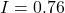A

Magnetic field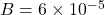T

Force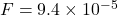N

Angle between wire and magnetic field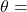46°

The magnetic force on the current carrying wire is given by,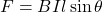Where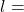length of wire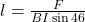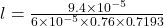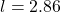m

Therefore, the length of the wire is 2.86 m